Algebra Fundamentals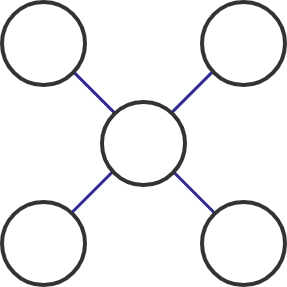Place the numbers $1$ to $5$ in the circles above, one number per circle, so that the sum of numbers on each of the two diagonal lines is $9.$

What number goes in the middle?

Magic Sum Puzzles

Puzzles like the previous one can be solved by just playing around with the numbers and figuring out where they fit.

However, rather than just doing arithmetic, we can think about the process involved in doing arithmetic—that is, do algebra—to make puzzles easier to solve. This also allows us to see the mechanics behind how they work.

In the next few questions, we'll examine various aspects of the problem below to see how algebraic thinking helps us solve problems like this.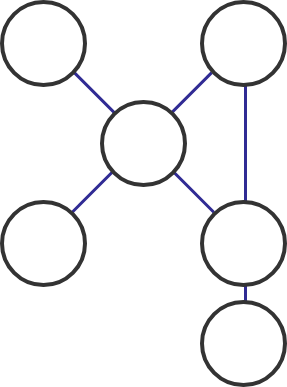Place all the numbers from $1$ to $6$ (one in each circle) so that the sum of numbers on each of the three marked lines comes out to be $12.$

Magic Sum Puzzles

We'll spend the next few questions considering the puzzle below:Place all the numbers from $1$ to $6$ (one in each circle) so that the sum of numbers on each of the three marked lines comes out to be $12.$

It can help to break the puzzle up into regions and compare them; one method is shown below. When we calculate all the sums described in the puzzle, what is the most important difference between the circles marked in red and those marked in green?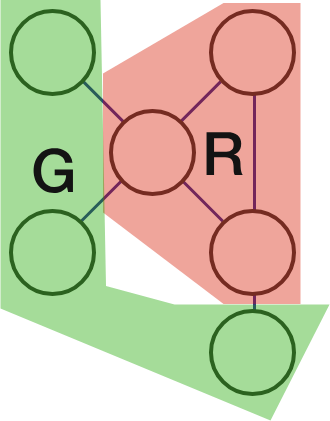Magic Sum PuzzlesPlace all the numbers from $1$ to $6$ (one in each circle) so that the sum of numbers on each of the three marked lines comes out to be $12.$

In order to analyze the puzzle, it helps to split the circles into an "inner section" where the sums overlap (marked red below) and an "outer section" (marked green below).If $R$ is the sum of numbers in the red section and $G$ is the sum of numbers in the green section, which equation is true? $($Remember we're using the numbers $1$ through $6.)$

Magic Sum Puzzles

Place all the numbers from $1$ to $6$ (one in each circle) so that the sum of numbers on each of the three marked lines comes out to be $12.$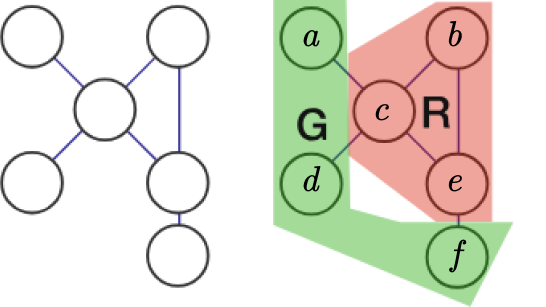When analyzing a puzzle with algebra, it helps to think of the same problem from multiple perspectives instead of thinking of all the numbers together.

As shown above, suppose we denote the values in these circles as $a,b,c,d,e,$ and $f.$

Because the sum of each of the three marked lines comes out to be $12,$ we can gather the three equations: \begin{aligned} a + c + e &= 12 \\ b + c + d &= 12 \\ b + e + f &= 12. \end{aligned} Adding these three equations together gives $(a + c + e) + (b + c + d) + (b + e + f) = 36 .$ If we rearrange the terms on the left-hand side, then which of the equations in the answer options can be formed?

Magic Sum Puzzles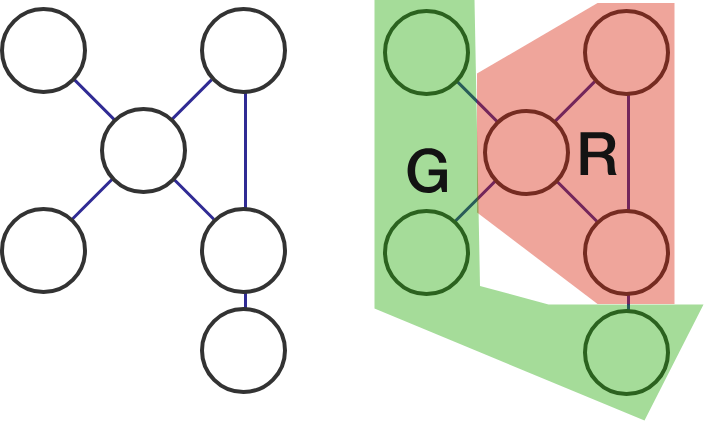Based on the previous problems, we now know that $R + G = 21$ and $2R + G = 36 .$ We can use algebra to combine these statements and figure out what $R$ and $G$ are.

What is $R?$ (Hint: Subtract the first equation from the second.)

Magic Sum PuzzlesPlace all the numbers from $1$ to $6$ (one in each circle) so that the sum of numbers on each of the three marked lines comes out to be $12.$

We have now learned that $R$ sums to $15.$ What number must go in the top left circle (in green) if $4$ is in the top right?

Magic Sum Puzzles

The algebra has led us to knowing where the sets $1, 2, 3$ and $4, 5, 6$ are.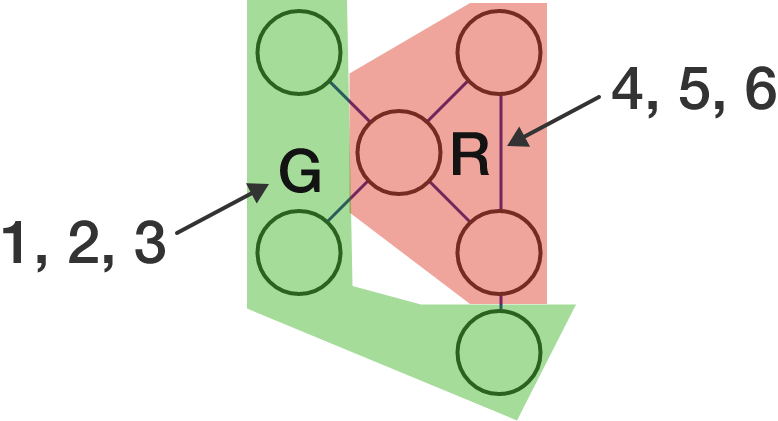Past this point, there are multiple options. With the last question we tried placing the $4$ in the upper right corner, but it can go anywhere in the red region; this leads to six different answers.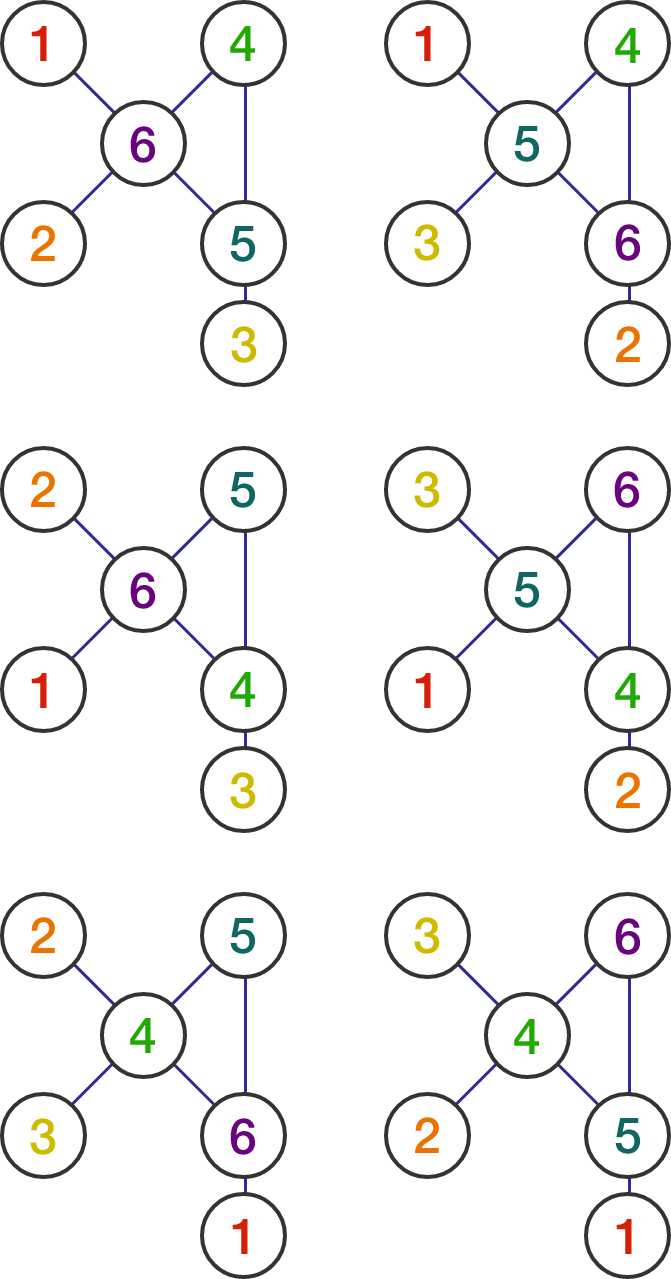While it'd be possible to find all the solutions above by guessing, algebra narrows down our search and makes it more systematic; it helps us to know we have all the solutions to the puzzle with greater confidence.

Magic Sum Puzzles

Let's change up the goal slightly and practice thinking algebraically!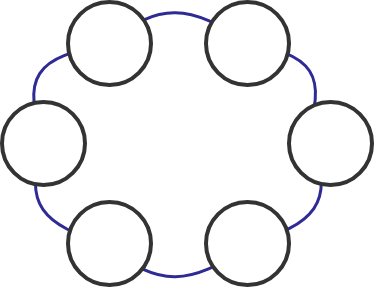Suppose you want to fill the puzzle above with the numbers $1$ through $6$ (one each circle) so that every set of three circles next to each other add up to the same sum.

Is this possible to do?

Magic Sum Puzzles

×